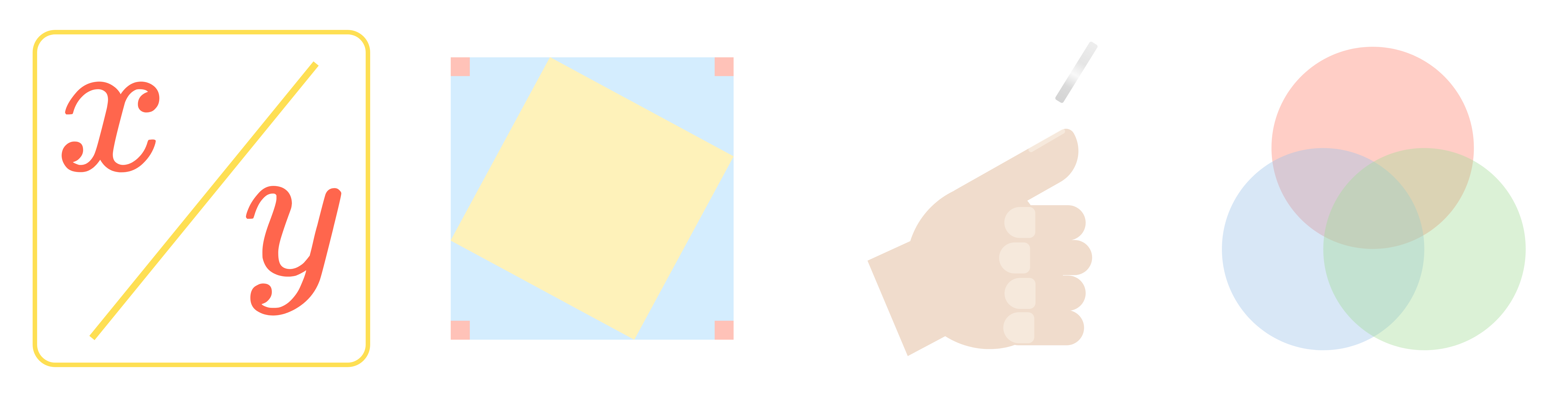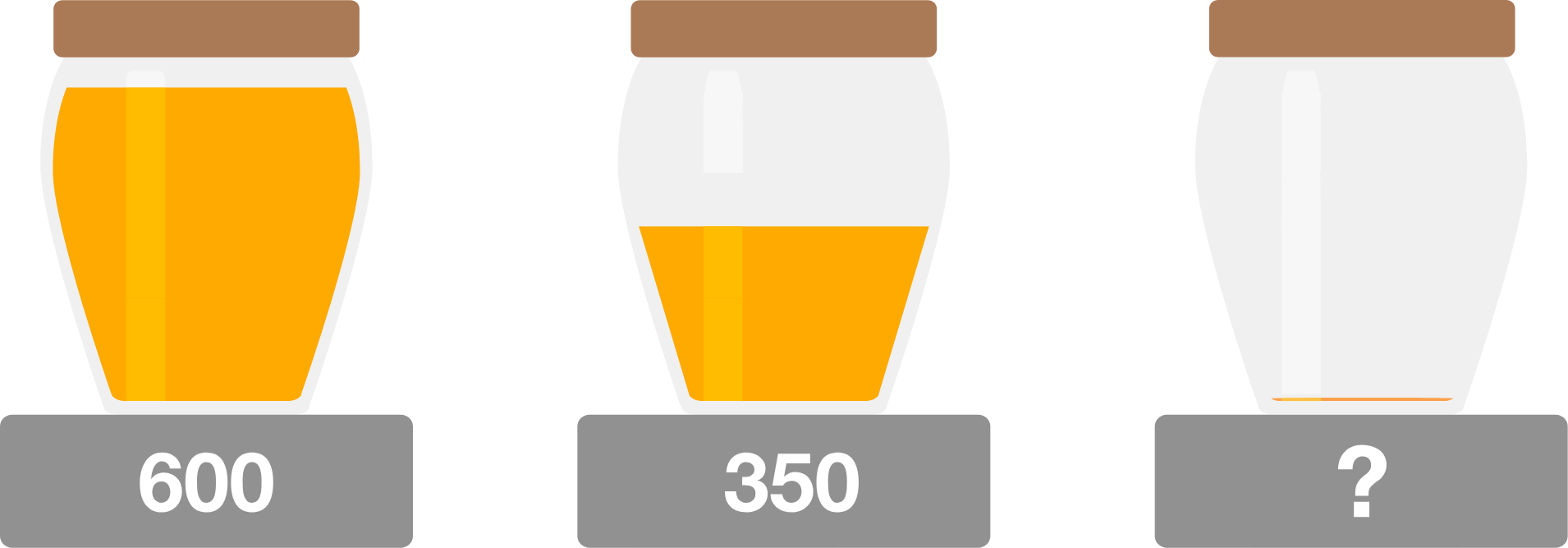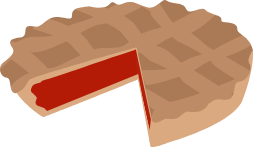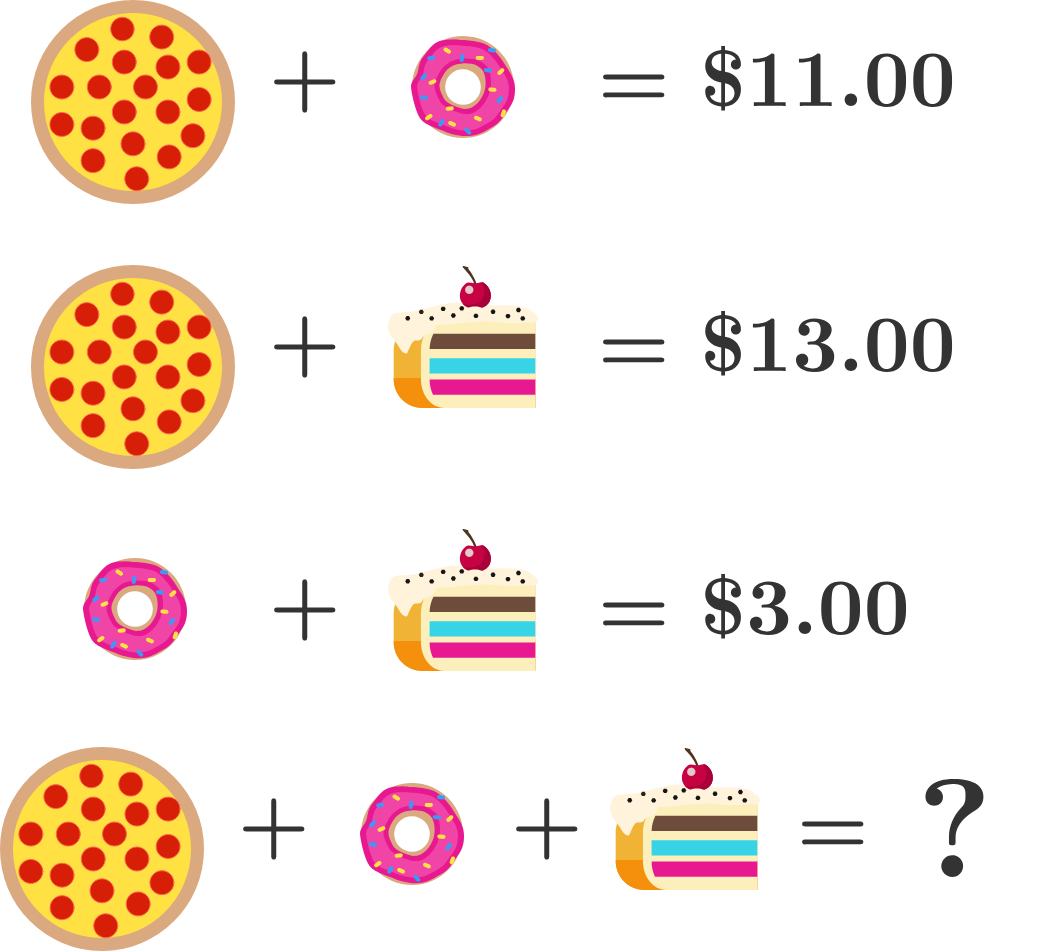### Contest Math I

In this course, we've sorted all of the math you need to learn for math competitions into four categories: algebra, geometry, combinatorics, and number theory!In the following problems, try to find ways to apply algebra that minimize the work needed to solve for each solution. Look for places where variables can be reused to represent multiple unknowns or where equations can be combined to save valuable time.

If you're looking to replicate competition conditions, time yourself going through this quiz and try to solve all 5 problems in ten to fifteen minutes.

# Algebra• The mass of a full jar of honey is 600 grams.
• With half of the honey, the total mass is 350 grams.

What is the mass of the empty jar in grams?

# Algebra

AlgebraIt takes 5 cooks 5 hours to bake 5 pies. Assuming no change of rate, how many hours will it take 10 cooks to bake 10 pies?

# Algebra

What is the cost of buying a pizza, a donut, and a piece of cake together?# Algebra

$\huge{\frac{{\color{#D61F06}1110}}{{\color{#D61F06}1111}}, \ \frac{{\color{#3D99F6}2221}}{{\color{#3D99F6}2223}}, \ \frac{{\color{#20A900}3331}}{{\color{#20A900}3334}}}$

Which is the largest?

# Algebra

Let $a,$ $b,$ $c,$ and $d$ be four numbers with $a < b$ and $c < d.$

The average of $a$ and $b$ is $c.$ The average of $c$ and $d$ is $b.$

If $d-a = 60,$ what is the value of $b-c?$

# Algebra

The algebra questions in math competitions are frequently either equation-focused or data-focused. We have units on each of these categories in this course, and you don't need to work through all of the units in this course in order, you can jump directly to whichever one you want to practice.

The unit on Equations will provide you with new tools to represent unknowns and develop your algebraic problem-solving intuition. To learn more, you can either explore what's in this unit or read through the Algebra: Equations Syllabus.

The unit on Data will show you ways to apply algebraic techniques to concrete sets of data and solve real-world applications. To learn more, you can either explore what's in this unit or read through the Algebra: Data Syllabus.

×